# Pie Charts

#### PIE CHARTS 1

PIE CHARTS 1

PIE CHARTS

A pie chart is a unique format of representing data in statistics. Here the independent variable is simply plotted around a circle. Or in other words a circle is broken up into different sectors by drawing radial lines from the centre and each component is either represented in terms of percentage or degrees to indicate the share of that component in the total. Pie Charts are primarily used to depict the relative sizes of the components. For example:

• The different costs incurred in the manufacturing of a product.

• Different sections in a hospital and number of doctors in each section giving their relative strengths,

• How popular are different breeds of dogs etc.

Here the table shows how many students got each grade in the recent test:

 A B C D 5 10 16 4

This can be represented in a pie chart as follows: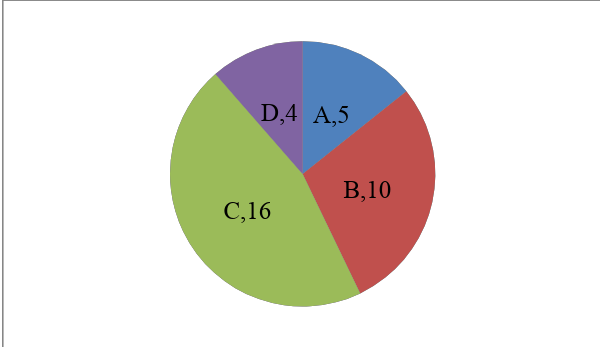Set-1

The following pie chart represents the breakup of Mohan’s monthly expenses.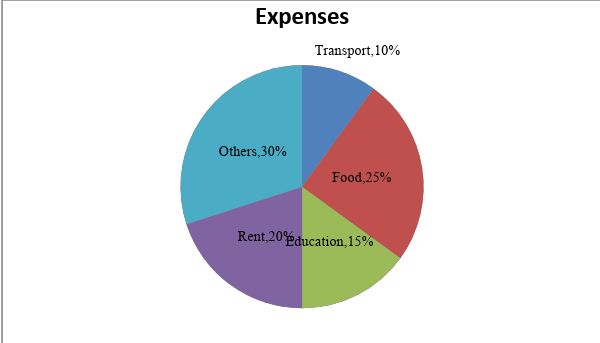1. If Mohan spent Rs 3000 more on food and education together than he spent on others, then find its monthly expenses.

(A)25000                        (B) 30000           (C) 20000          (D) 15000

Solution: (B)

Percentage of his expenditure on food and education together is = 25+15 = 40

Percentage of his expenditure on others = 30

Given that, (40 %- 30%) = 3000

Monthly expenses = 3000/0.1 = 30000

1. If Mohan increased his savings, which is currently 20 % of income by 30% and reduced his expenses by 30 %, then his savings would be what percentage of his expanses?

(A) 35 %            (B) 45 %        (C) 41.27%         (D) 46.42 %

Solution :  (D)

Assume, his monthly income is Rs ‘x’

Initially savings,  10x/100

New Savings,Initially expenditure, =New expenditure, =Require percentage == 46.42 %

1. Mohan spent 20 % of his expenditure on others on clothes which is equal to half of expenditure on transportation. If the expenditure on transportation is 6000 rs. Find his Expenditure on rent.

(A10000            (B) 5000         (C) 3000           (D) 6000

Solution: (A)

Expenditure on clothes = 20 % of 30 % = 6 % = 3000

Total expenditure is : 50000

Expenditure on rent = 20 % of 50000 = 10000

1. Find the angle made by the expenditure on rent and transportation put together.

(A180            (B) 120         (C) 108         (D) 60

Solution : (C)

Required angle is= 108

1. As prices dropped Mohan’s expenditure on clothes dropped by 10 %. As a result of this, his expenditure on others decreases from 10000 to 9500. What percentage of his expenditure on others was spent on clothes?

(A20 %            (B) 50 %        (C) 40 %        (D) 60 %

Solution: (B)

Decrease in expenditure on others = 500 which is 10% of total expenditure on clothes

Total expenditure on clothes = 500 * 10/100 = 5000

Required percentage = (5000/ 10000) * 100 = 50 %

Set 2:

The following chart represents the number of votes cast for different political parties in an election in the year 2014. The total number of votes cast in that election is 2, 50, 000.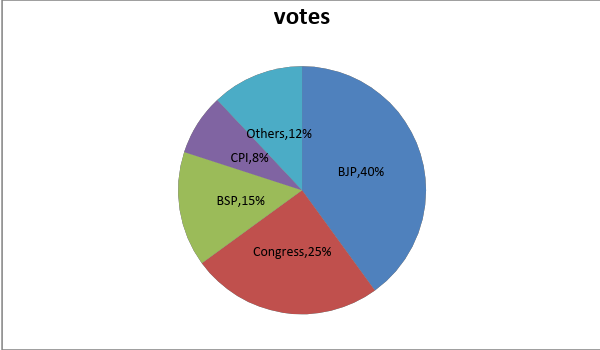1. If the number of votes cast for the BJP is 25% more than that in the previous election, then what is the difference between number of votes cast for BJP in this election and previous one?

(A10000        (B) 80000        (C) 20000    (D) 25000

Solution; (C)

Votes cast for BJP in this year = 40% of  250000 = 100000

If votes cast for BJP in previous election is ‘x’

Then, 1.25 x = 100000

‘x’ = 80000

The required difference = 100000- 80000 = 20000

1. If the number of votes cast for BSP in 2014 is 25 % less that in the previous election, which was 20 % of the total votes cast in that year, then how many votes were cast in total in the previous election?

(A250000        (B) 200000        (C) 300000        (D) 2750000

Solution : (A)

In 2014, BSP got = 15 % of 250000 = 37500

Suppose vote cast for BSP in the previous election is  ‘x’

Given that, 0.75x = 37500

‘x’ = 50000 which is 20% of total vote caste in previous election.

Therefore, total vote cast 250000

1. If 10 % of total votes cast for BJP in 2014 is equal to votes cast for congress and BSP together in the previous election. BSP got 35 % of vote cast for BSP and congress together in the previous election. Calculate Number of votes cast for congress in previous election.

(A100000        (B) 35000        (C) 65000        (D) 6500

Solution : (D)

Votes cast for BJP in this year (2014) = 40% of  250000 = 100000

10 % of 100000 = 10000.

Congress got 65 %  votes in the previous election = 65 % of 10000 = 6500

1. By what percentage is the total votes cast for the Congress party more than that for others?

(A50 %         (B) 54 %        (C) 48%        (D) 108.33%

Solution : (D)

Required percentage  13/12 * 100 = 108.33

1. If out of total votes cast , 20000 votes were found to be invalid, BSP got 5000 invalid votes ,then what % of total  valid votes cast for BSP ?

(A15.20        (B) 13.15        (C) 14.13            (D)  14.50

Solution: (C)

Total valid votes = 250000-20000 = 230000

Total votes cast for BSP = 15% of 250000 = 37500

Valid votes cast for BSP  37500- 5000 = 32500

Required percentage = (32500/ 230000)*100  = 14.13

Set- 3

The first Pie chart gives the break- up of the number of students who appeared for an examination from six states- A, B, C, D, E, and F. The second pie chart gives the breakup of the students who qualified the exam.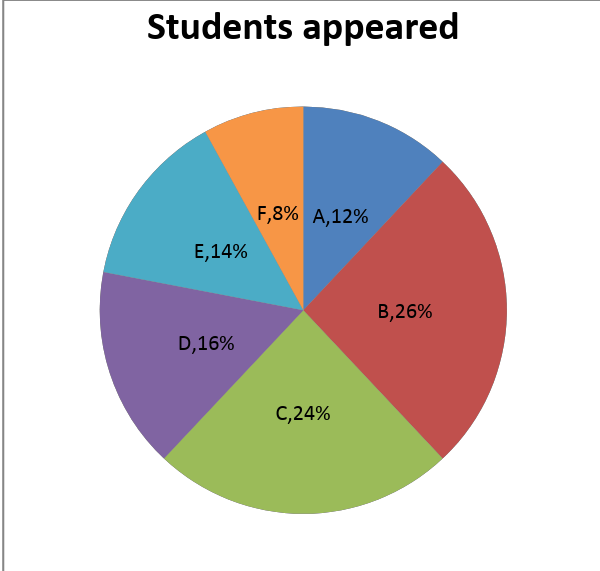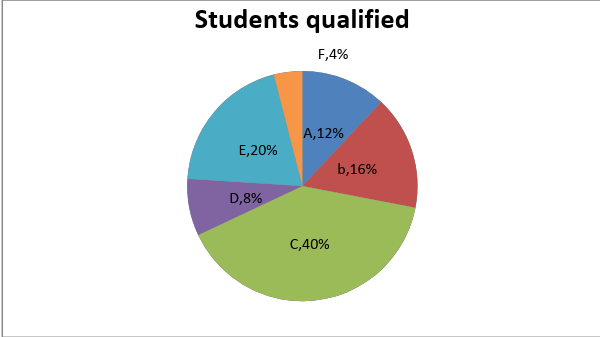1. If 50 % of the students from the state ‘A’ qualified , what percentage of students qualified from state ‘C’?

(A83.34 %                (B) 50 %        (C)  40.34 %        (D) 45.67 %

Solution : (A)

Suppose, the total number of students who appeared be 100x and qualified be 100y.

Given that, percentage of students qualified from ‘A’ =Therefore,Percentage of students qualified from ‘C’ =1. What is the least number of students who did not qualify from all the states together?

(A25            (B) 60            (C) 75             (D) 120

Solution: (A)

The number of students who don’t qualify = 100x- 100y

For least, x = 0.50 and y = 0.25 (number of students must be integer)

Answer -: 100*0.5 – 100*.25 = 25

1. If the number of students who qualified from the state ‘A’ = 15, then what is the least number of students who did not qualify from all the states together?

(A25        (B) 40        (C) 125        (D) Cannot be determined

Solution: (C)

Given that, 12y = 15

‘y’  = 1.25

For 100x – 100y to be least, ‘x’ must be 2.5

Therefore, 100x-100y = 125

1. If number of the students who did not qualify from state ‘A’ are 48, then what is the total number of students who did not qualify from all the states together?

(A350             (B) 400              (C)  450        (D) Data insufficient

Solution: (B)

Give that, 12x-12y = 48

(x-y) = 4

Therefore, (100x -100y) = 100*4 = 400

1. Calculate percentage of students who qualified from state ‘A’ to state ‘D and ‘C” put together?

1. 15 %            (B) 25 %            (C) 40 %        (D) 48 %

Solution: (B)

Required percentage = 12/48 * 100 = 25 %

Set-4

The pie chart gives the breakup of runs scored in a match by all five players who batted for a team in a tournament. The table gives the share of the number of fours and sixes scored by the batsman.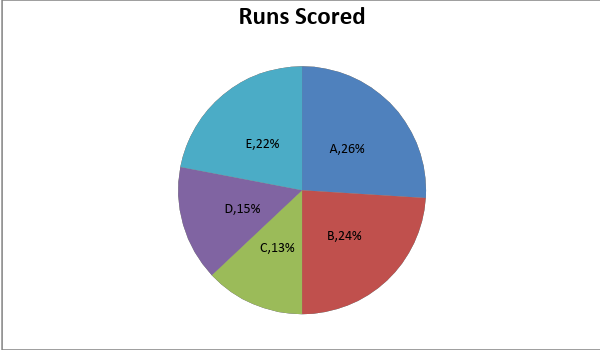Player 4’s 6’s A 13.33 12.5 B 20.00 25 C 33.33 0 D 6.67 37.5 E 26.67 25

1. How many fours (4’s) did the team score in total?

(A10            (B)15            (C) 18            (D)  16

Solution: (B)

Since, Player ’D’ scored 6.67 % of the total number of 4’s. Therefore, the number of 4’s the team scored is at least 15 (to be an integer)

The minimum number of sixes team scored is = 8

1. How many runs did player ‘A’ Score?

(A48             (B) 52            (C)   30            (D) 44

Solution: (B)

The total run scored by players in 4’s and 6’s are:

 Player 4’s 6’s Total Runs (4+6) A 8 6 14 B 12 12 24 C 20 0 20 D 4 18 22 E 16 12 28

As ‘C’ scored 13 % of the runs scored, the total runs scored by team must be at least 200.

1. Who among the players scored maximum runs through 4’s or 6’s?

(AA            (B) B            (C) C            (D) E

Solution: (C)

 Player Total Runs scored 4’s 6’s Total Runs (4+6) A 52 8 6 14 B 48 12 12 24 C 26 20 0 20 D 30 4 18 22 E 44 16 12 28

1. How many Runs did the five players together score?

(A200            (B) 150            (C) 300        (D) 250

Solution: (A)

1. Calculate percentage of runs scored by ‘A’ through  4’s and 6’s to the total runs scored by ‘C’ and ‘D’ together?

(A14 %